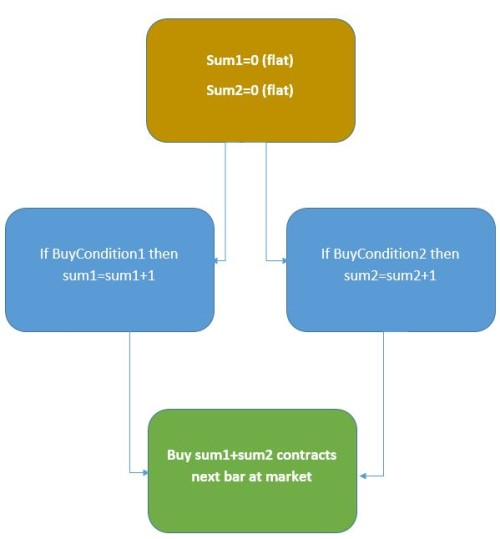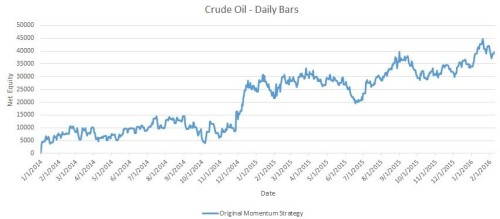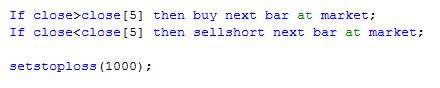• You are here:
• Home »Trading Multiple Strategies With The Same Instrument – Part 2 [Updated For 2021]

In the previous article, I discussed the need for trading multiple strategies with the same instrument in the same account. This type of capability is very important to a trader who wishes to trade a diversified portfolio, while at the same time efficiently managing margin. Unfortunately, one of the limitations of Tradestation, my trading platform of choice, is that this capability is very tough to achieve.

As you will recall from the earlier article, we discussed six different options to trade multiple strategies with the same instrument. In this article, we will layout the process and the code to create a summing strategy, which was Option number 6. The steps to take in such an approach are as follows:

1. Create a typical strategy, using standard Tradestation reserved words such as “setstoploss”
2. Transform this typical strategy into a “summing” strategy. An example of how a summing strategy works is shown in Figure 1
3. Check that summing strategy matches the original strategy
4. Combine multiple summing strategies into a master strategyFigure 1 – Example of a Summing Strategy

Today’s article will focus on the first three steps, and the final article in this series will focus on the fourth step.

For the baseline normal strategy we will just use the simple strategy described in part 1 – a simple momentum strategy with a stoploss. This is typical of a very simple strategy. We will not include a profit target, but the conversion process from a typical strategy to a summing strategy could easily handle this. This simple strategy produces the equity curve shown in Figure 2.Figure 2 – Original Momentum Strategy Equity Curve

This is the curve we will try to mimic with the summing strategy. It is important to note that we say “mimic” and not “duplicate.” For example, for various reasons it is not possible to correctly use Tradestation stoploss functions when combining multiple strategies. So, we will attempt to get close to the original strategy, but the summing strategy performance may be better, or it may be worse. That is the price we must pay to reach the end objective.

In looking at the original strategy, the entry – a simple “buy the next bar at market” – should be fairly easy to implement. The stop loss order will be a bit more difficult. This is because the stop loss is active. The whole bar, and the new strategy will only act at end of bars.

To keep the conversion simple, I am going to use “sell next bar at market” to replicate the stops. This will mean there is no stop in the market when you actually trade it live. This could be a deal breaker for some people, but in reality the difference between using actual stop losses and simply exiting the next bar at market is not troublesome in most strategies. If it is significant, the pseudo stop price can be adjusted to more closely match the original strategy. Again, though, we are giving up the power of the stoploss function to be able to more easily create the summing strategy.

Figure 3 shows the original strategy created for step 1.Figure 3 – Original Strategy Code, Momentum Strategy

Below shows the summing strategy created for step 2.

{TRY TO MIMIC THIS

If close>close then buy next bar at market;
If close<close then sellshort next bar at market;

setstoploss(1000);}

input: stopl(1000),ncons01(1);

var: PositionAtEnd01(0),LongEntry(0),ShortEntry(0);

//this is the current position we should be at the start of the bar
//PositionAtEnd01;

//Entry rule

If close>close then begin
PositionAtEnd01=1; //should be long at open of next bar
If PositionAtEnd01<>1 then LongEntry=Close-stopl/BigPointValue; //reset longentry only if New trade
End;

// If Condition2 then begin Condition2 is your sellshort signal
If close<close then begin
PositionAtEnd01=-1; //should be short at open of next bar
If PositionAtEnd01<>-1 then ShortEntry=Close+stopl/BigPointValue; //reset shortentry only if New trade
End;

//now check current position and see if pseudo stop was hit- if it was, then make sure position at open of next bar is flat
If (positionAtEnd01=1 ) and Close<=LongEntry then PositionAtEnd01=0;
If (positionAtEnd01=-1 ) and Close>=ShortEntry then PositionAtEnd01=0;

//now at this point, we have three possibilities
//PositionAtEnd01=1 means at open of next bar, we should be LONG ncons01 contracts
//PositionAtEnd01=-1 means at open of next bar, we should be SHORT ncons01 contracts
//PositionAtEnd01=0 means at open of next bar, we should be FLAT

//***********************************************
//we have to figure out how many long or short we should be

totalcons=0;
If PositionAtEnd01=1 then totalcons=ncons01;
If PositionAtEnd01=-1 then totalcons=-ncons01;

//so now totalcons should be the final position, after all is said and done

CurrentCons=CurrentContracts*Marketposition; //this is our current posirion at the close of the current bar

//print(date," ",totalcons," ",currentcons);

//first figure out how many contracts to buy or sell to get to right NET LONG position

If TotalCons>0 then begin
If currentcons>0 then begin
//we are long, but have to decrease our long position (but still stay long)
if totalcons<currentcons then begin Sell currentcontracts-totalcons contracts total next bar at market;
end;

end;

end;

//next figure out how many contracts to buy or sell to get to right NET SHORT position
constosellshort=0;

If TotalCons<0 then begin
If currentcons>=0 then constosellshort= -totalcons;

If currentcons<0 then begin
if totalcons>currentcons then begin
//we are short, but have to decrease our short position (but still stay Short)
Buytocover (currentcontracts+totalcons) contracts total next bar at market;
end;

If totalcons<currentcons then constosellshort= -(totalcons-currentcons) ;
end;

sellshort constosellshort contracts next bar at market;
end;

//if we should be flat overall, then exit all existing contracts

If TotalCons=0 then Sell All contracts next bar at market;
If TotalCons=0 then BuyToCover All contracts next bar at market;

By examining the code, it should be pretty clear what is going on – order statements like “buy next bar at market” are replaced with counters/summing variables, and at the end of the code, the counters/summing variables are converted back into buy and sell statements.

Some of the code for the bottom section looks overly complex, and it is that way by necessity. Tradestation has some weird quirks with order placement that must be accounted for. For example, let’s say you have a strategy with two separate buy statements triggered, corresponding to different conditions. So, now you’d be long two contracts, one from each entry. If you later wanted to exit (sell) only one of these contracts, a statement such as “sell 1 contract next bar at market;” will actually sell one contract from each entry.

So, the architecture of Tradestation makes keeping track of orders and where they come from pretty difficult. Thus, the code at the bottom of the summing strategy is complicated. In the end, the accuracy of this code can be determined by comparing the individual summing strategies with the single “master” summing strategy. That will be demonstrated in part 3.

Figure 4 shows the equity curve for each of the strategies. As you can see, the results are fairly similar – close enough for us to accept the summing strategy of Figure 4 in lieu of the original strategy of Figure 3.Figure 4 – Comparison of Original and Summing Strategies

Eventually, as the trader gets more comfortable with this conversion process, he or she can start out from the beginning with the summing strategy, bypassing the original strategy completely. If this strategy produces acceptable performance results, there really is no need to create the original strategy. A sample template for an individual summing strategy is shown in the code at the bottom of the article.

Now that we can convert a “normal” strategy into a format that can work with a summing strategy, we can combine multiple strategies into one large strategy. That will be tackled in the final part of this article series.